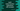# Find the maximum of n values using max() in Swift## Swift max() function explanation with example:

In this post, we will learn how to use the max function with examples. max() function can be used to find the maximum of n different values. We will use this function with numbers and with custom class objects.

### Definition:

max() is defined as below:

``func max<T>(_ a: T, _ b: T, _ c: T, _ rest: T...) -> T``

Where,

• T is a Comparable
• a is the first value to compare
• b is the second value to compare
• c is the third value to compare
• rest is zero or more values to compare

It returns the largest value among the provided values.

It returns the first element if two or more elements are equal and both are largest.

### Example program:

Let’s take a look at the below program:

``````print(max(1, 2))
print(max(1, 2, 3))
print(max(1, 2, 3, 4, 5, 6, 7, 8, 9, 0))``````

This program is trying to find the largest value among multiple numbers.This program will print the below output:

``````2
3
9``````

### Example with custom objects:

We can also use custom objects with max() function. For that, we need the class as subclass of Comparable. For example:

``````class Result: Comparable{
var name: String
var marks: Int

init(name: String, marks: Int) {
self.name = name
self.marks = marks
}

static func < (lhs: Result, rhs: Result) -> Bool {
return lhs.marks < rhs.marks
}

static func == (lhs: Result, rhs: Result) -> Bool {
return lhs.marks == rhs.marks
}

func toString(){
print("Name: \(self.name), Marks: \(self.marks)")
}
}

let result1 = Result(name: "Albert", marks: 20)
let result2 = Result(name: "Oliver", marks: 18)
let result3 = Result(name: "Noah", marks: 17)

max(result1, result2).toString()
max(result2, result3).toString()
max(result1, result2, result3).toString()``````

Here,

• Class Result is a subclass of Comparable. It can keep one string name and one integer marks.
• It implements the < function and == function.
• Three objects are created result1, result2 and result3. All are Result objects. We are calling max() function with these objects to find the maximum value. The toString() method prints the content of each objects.

If you run this program, it will print the below output:

``````Name: Albert, Marks: 20
Name: Oliver, Marks: 18
Name: Albert, Marks: 20``````